Research papers and code for "Ke Xu":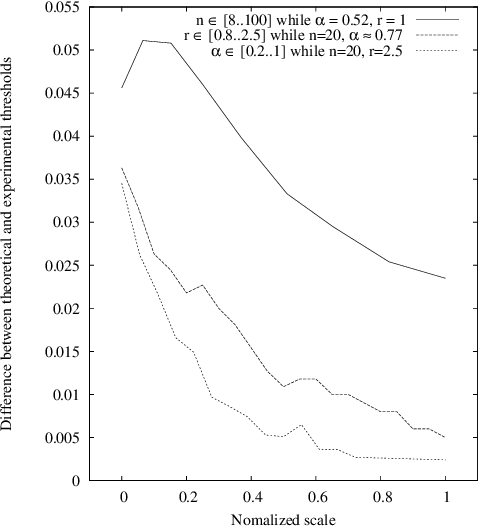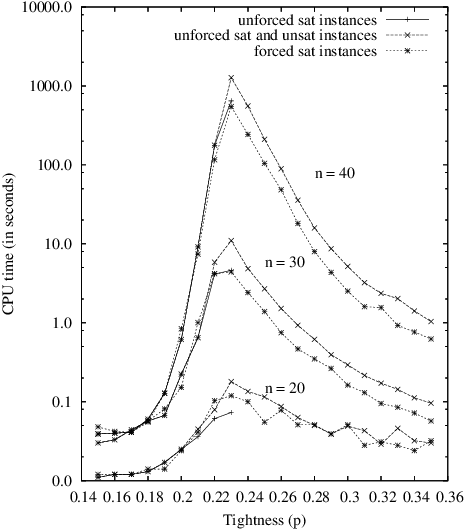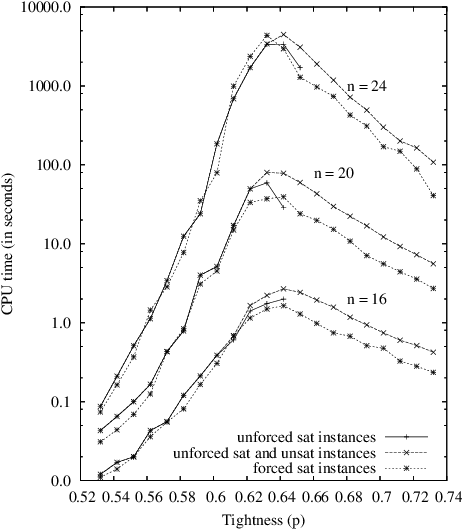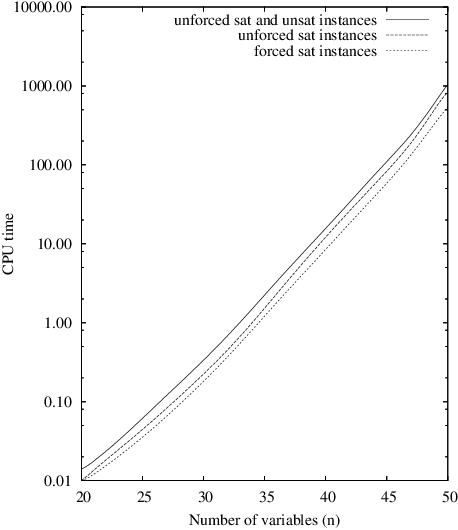In this paper, we try to further demonstrate that the models of random CSP instances proposed by [Xu and Li, 2000; 2003] are of theoretical and practical interest. Indeed, these models, called RB and RD, present several nice features. First, it is quite easy to generate random instances of any arity since no particular structure has to be integrated, or property enforced, in such instances. Then, the existence of an asymptotic phase transition can be guaranteed while applying a limited restriction on domain size and on constraint tightness. In that case, a threshold point can be precisely located and all instances have the guarantee to be hard at the threshold, i.e., to have an exponential tree-resolution complexity. Next, a formal analysis shows that it is possible to generate forced satisfiable instances whose hardness is similar to unforced satisfiable ones. This analysis is supported by some representative results taken from an intensive experimentation that we have carried out, using complete and incomplete search methods.

* Proc. of 19th IJCAI, pp.337-342, Edinburgh, Scotland, 2005. For more information, please click http://www.nlsde.buaa.edu.cn/~kexu/papers/ijcai05-abstract.htm
Click to Read Paper and Get Code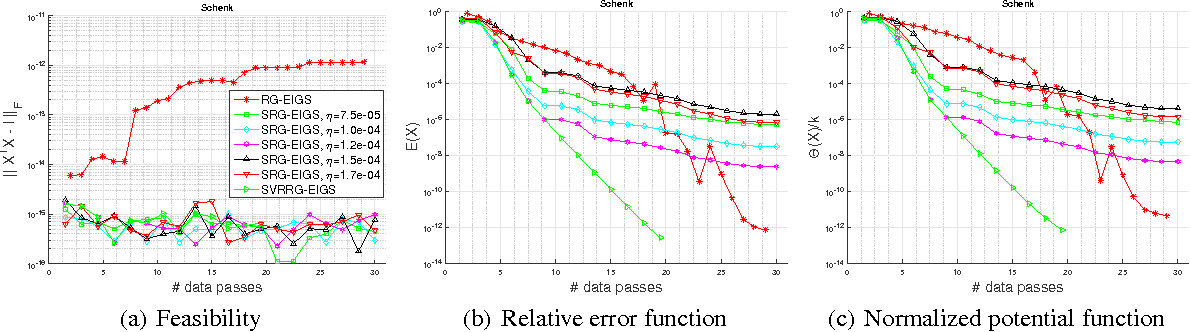We study the stochastic Riemannian gradient algorithm for matrix eigen-decomposition. The state-of-the-art stochastic Riemannian algorithm requires the learning rate to decay to zero and thus suffers from slow convergence and sub-optimal solutions. In this paper, we address this issue by deploying the variance reduction (VR) technique of stochastic gradient descent (SGD). The technique was originally developed to solve convex problems in the Euclidean space. We generalize it to Riemannian manifolds and realize it to solve the non-convex eigen-decomposition problem. We are the first to propose and analyze the generalization of SVRG to Riemannian manifolds. Specifically, we propose the general variance reduction form, SVRRG, in the framework of the stochastic Riemannian gradient optimization. It's then specialized to the problem with eigensolvers and induces the SVRRG-EIGS algorithm. We provide a novel and elegant theoretical analysis on this algorithm. The theory shows that a fixed learning rate can be used in the Riemannian setting with an exponential global convergence rate guaranteed. The theoretical results make a significant improvement over existing studies, with the effectiveness empirically verified.

* Under review. Supplementary material included in the paper as well
Click to Read Paper and Get Code
This paper first analyzes the resolution complexity of two random CSP models (i.e. Model RB/RD) for which we can establish the existence of phase transitions and identify the threshold points exactly. By encoding CSPs into CNF formulas, it is proved that almost all instances of Model RB/RD have no tree-like resolution proofs of less than exponential size. Thus, we not only introduce new families of CNF formulas hard for resolution, which is a central task of Proof-Complexity theory, but also propose models with both many hard instances and exact phase transitions. Then, the implications of such models are addressed. It is shown both theoretically and experimentally that an application of Model RB/RD might be in the generation of hard satisfiable instances, which is not only of practical importance but also related to some open problems in cryptography such as generating one-way functions. Subsequently, a further theoretical support for the generation method is shown by establishing exponential lower bounds on the complexity of solving random satisfiable and forced satisfiable instances of RB/RD near the threshold. Finally, conclusions are presented, as well as a detailed comparison of Model RB/RD with the Hamiltonian cycle problem and random 3-SAT, which, respectively, exhibit three different kinds of phase transition behavior in NP-complete problems.

* 19 pages, corrected mistakes in Theorems 5 and 6
Click to Read Paper and Get Code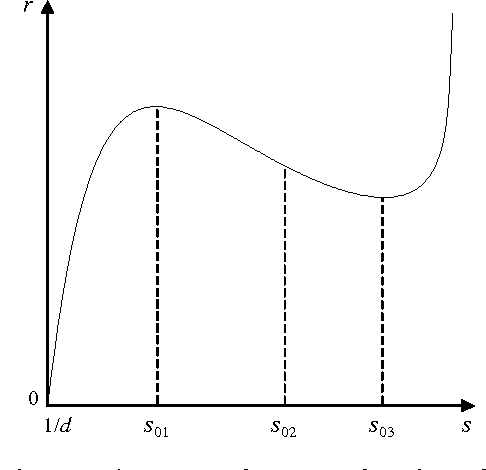To study the structure of solutions for random k-SAT and random CSPs, this paper introduces the concept of average similarity degree to characterize how solutions are similar to each other. It is proved that under certain conditions, as r (i.e. the ratio of constraints to variables) increases, the limit of average similarity degree when the number of variables approaches infinity exhibits phase transitions at a threshold point, shifting from a smaller value to a larger value abruptly. For random k-SAT this phenomenon will occur when k>4 . It is further shown that this threshold point is also a singular point with respect to r in the asymptotic estimate of the second moment of the number of solutions. Finally, we discuss how this work is helpful to understand the hardness of solving random instances and a possible application of it to the design of search algorithms.

* Discrete Applied Mathematics, 136(2004):125-149.
* 22 pages, the final version to appear in Discrete Applied Mathematics
Click to Read Paper and Get Code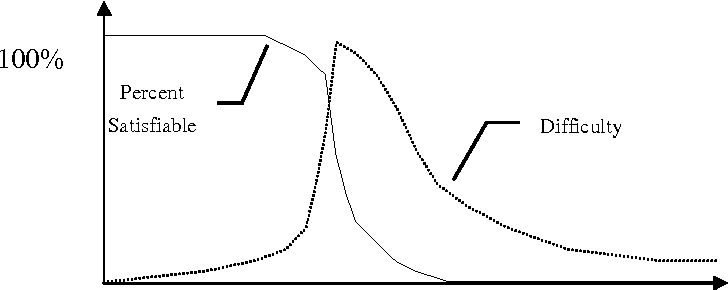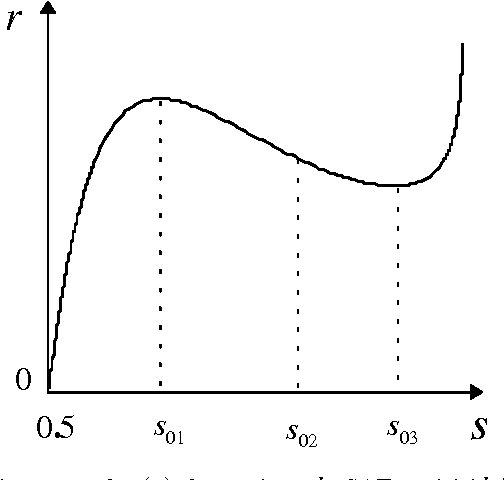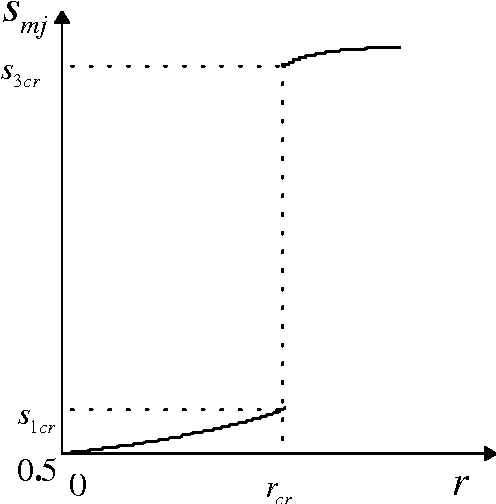Phase transition is an important feature of SAT problem. For random k-SAT model, it is proved that as r (ratio of clauses to variables) increases, the structure of solutions will undergo a sudden change like satisfiability phase transition when r reaches a threshold point. This phenomenon shows that the satisfying truth assignments suddenly shift from being relatively different from each other to being very similar to each other.

* The SAT Phase Transition. Science in China, Series E, 42(5):494-501, 1999
* 13 pages, 3 figures
Click to Read Paper and Get Code
In this paper we propose a random CSP model, called Model GB, which is a natural generalization of standard Model B. It is proved that Model GB in which each constraint is easy to satisfy exhibits non-trivial behaviour (not trivially satisfiable or unsatisfiable) as the number of variables approaches infinity. A detailed analysis to obtain an asymptotic estimate (good to 1+o(1)) of the average number of nodes in a search tree used by the backtracking algorithm on Model GB is also presented. It is shown that the average number of nodes required for finding all solutions or proving that no solution exists grows exponentially with the number of variables. So this model might be an interesting distribution for studying the nature of hard instances and evaluating the performance of CSP algorithms. In addition, we further investigate the behaviour of the average number of nodes as r (the ratio of constraints to variables) varies. The results indicate that as r increases, random CSP instances get easier and easier to solve, and the base for the average number of nodes that is exponential in r tends to 1 as r approaches infinity. Therefore, although the average number of nodes used by the backtracking algorithm on random CSP is exponential, many CSP instances will be very easy to solve when r is sufficiently large.

* Annals of Mathematics and Artificial Intelligence, 33:21-37, 2001.
* 20 pages, submitted to Annals of Mathematics and Artificial Intelligence
Click to Read Paper and Get Code
In this paper we propose a new type of random CSP model, called Model RB, which is a revision to the standard Model B. It is proved that phase transitions from a region where almost all problems are satisfiable to a region where almost all problems are unsatisfiable do exist for Model RB as the number of variables approaches infinity. Moreover, the critical values at which the phase transitions occur are also known exactly. By relating the hardness of Model RB to Model B, it is shown that there exist a lot of hard instances in Model RB.

* Journal of Artificial Intelligence Research, Vol 12, (2000), 93-103.
* See http://www.jair.org/ for any accompanying files
Click to Read Paper and Get Code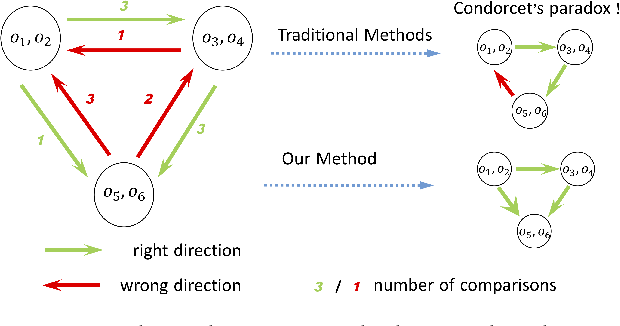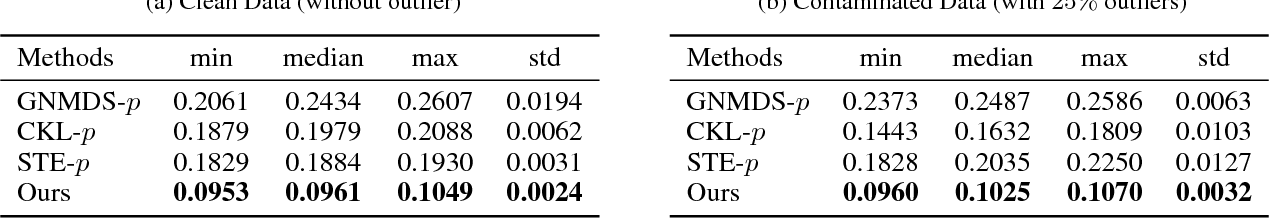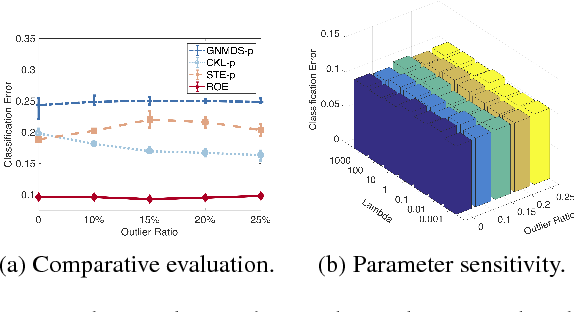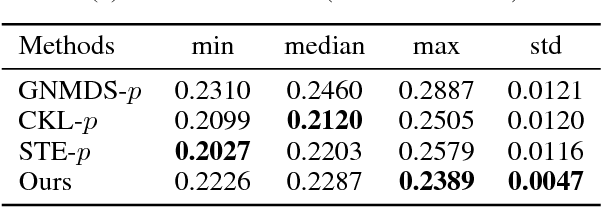Existing ordinal embedding methods usually follow a two-stage routine: outlier detection is first employed to pick out the inconsistent comparisons; then an embedding is learned from the clean data. However, learning in a multi-stage manner is well-known to suffer from sub-optimal solutions. In this paper, we propose a unified framework to jointly identify the contaminated comparisons and derive reliable embeddings. The merits of our method are three-fold: (1) By virtue of the proposed unified framework, the sub-optimality of traditional methods is largely alleviated; (2) The proposed method is aware of global inconsistency by minimizing a corresponding cost, while traditional methods only involve local inconsistency; (3) Instead of considering the nuclear norm heuristics, we adopt an exact solution for rank equality constraint. Our studies are supported by experiments with both simulated examples and real-world data. The proposed framework provides us a promising tool for robust ordinal embedding from the contaminated comparisons.

* Accepted by AAAI 2019
Click to Read Paper and Get Code
The growing explosion in the use of surveillance cameras in public security highlights the importance of vehicle search from large-scale image databases. Precise vehicle search, aiming at finding out all instances for a given query vehicle image, is a challenging task as different vehicles will look very similar to each other if they share same visual attributes. To address this problem, we propose the Repression Network (RepNet), a novel multi-task learning framework, to learn discriminative features for each vehicle image from both coarse-grained and detailed level simultaneously. Besides, benefited from the satisfactory accuracy of attribute classification, a bucket search method is proposed to reduce the retrieval time while still maintaining competitive performance. We conduct extensive experiments on the revised VehcileID dataset. Experimental results show that our RepNet achieves the state-of-the-art performance and the bucket search method can reduce the retrieval time by about 24 times.

Click to Read Paper and Get Code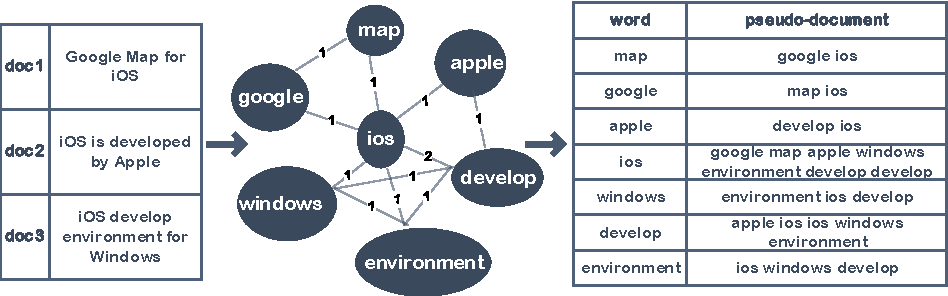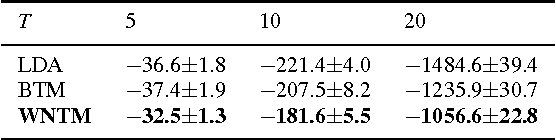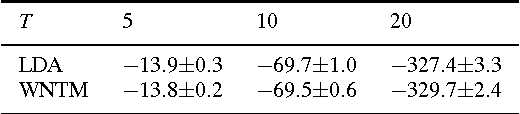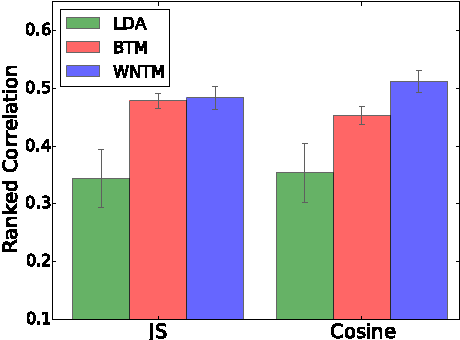The short text has been the prevalent format for information of Internet in recent decades, especially with the development of online social media, whose millions of users generate a vast number of short messages everyday. Although sophisticated signals delivered by the short text make it a promising source for topic modeling, its extreme sparsity and imbalance brings unprecedented challenges to conventional topic models like LDA and its variants. Aiming at presenting a simple but general solution for topic modeling in short texts, we present a word co-occurrence network based model named WNTM to tackle the sparsity and imbalance simultaneously. Different from previous approaches, WNTM models the distribution over topics for each word instead of learning topics for each document, which successfully enhance the semantic density of data space without importing too much time or space complexity. Meanwhile, the rich contextual information preserved in the word-word space also guarantees its sensitivity in identifying rare topics with convincing quality. Furthermore, employing the same Gibbs sampling with LDA makes WNTM easily to be extended to various application scenarios. Extensive validations on both short and normal texts testify the outperformance of WNTM as compared to baseline methods. And finally we also demonstrate its potential in precisely discovering newly emerging topics or unexpected events in Weibo at pretty early stages.

Click to Read Paper and Get Code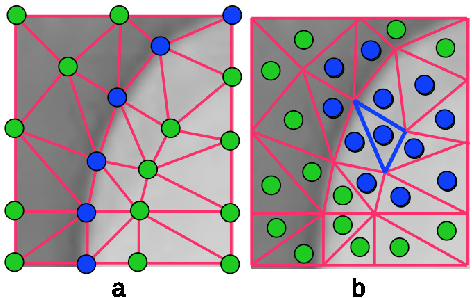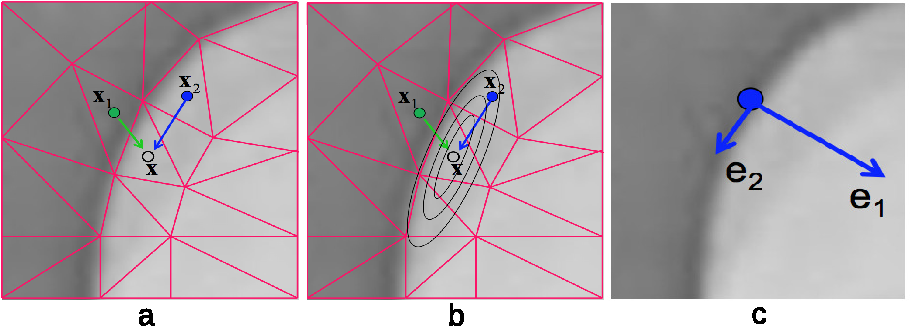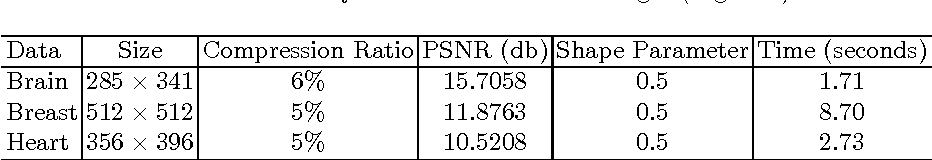The triangulation of images has become an active research area in recent years for its compressive representation and ease of image processing and visualization. However, little work has been done on how to faithfully recover image intensities from a triangulated mesh of an image, a process also known as image restoration or decoding from meshes. The existing methods such as linear interpolation, least-square interpolation, or interpolation based on radial basis functions (RBFs) work to some extent, but often yield blurred features (edges, corners, etc.). The main reason for this problem is due to the isotropically-defined Euclidean distance that is taken into consideration in these methods, without considering the anisotropicity of feature intensities in an image. Moreover, most existing methods use intensities defined at mesh nodes whose intensities are often ambiguously defined on or near image edges (or feature boundaries). In the current paper, a new method of restoring an image from its triangulation representation is proposed, by utilizing anisotropic radial basis functions (ARBFs). This method considers not only the geometrical (Euclidean) distances but also the local feature orientations (anisotropic intensities). Additionally, this method is based on the intensities of mesh faces instead of mesh nodes and thus provides a more robust restoration. The two strategies together guarantee excellent feature-preserving restoration of an image with arbitrary super-resolutions from its triangulation representation, as demonstrated by various experiments provided in the paper.

Click to Read Paper and Get Code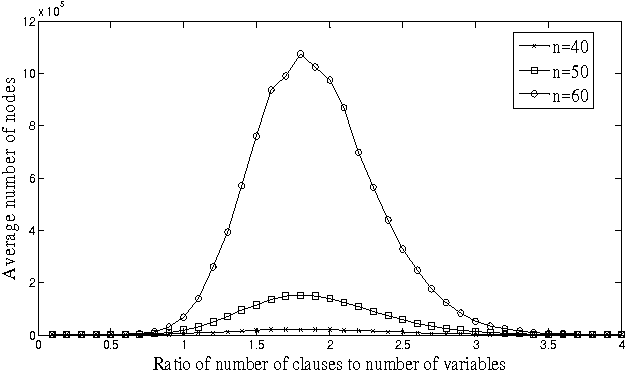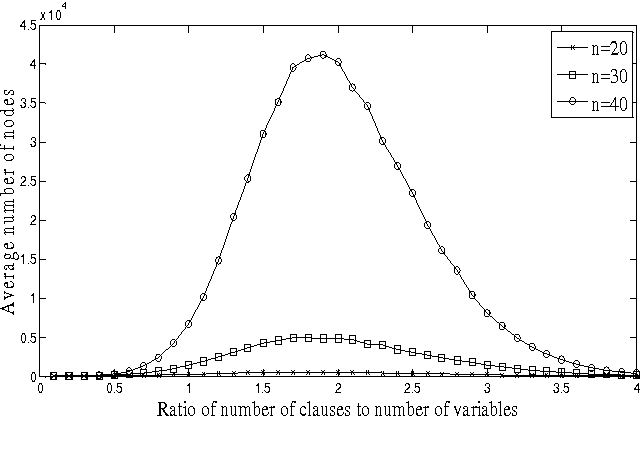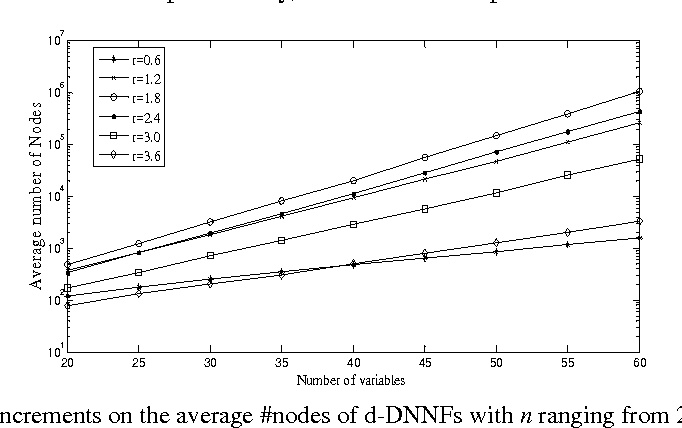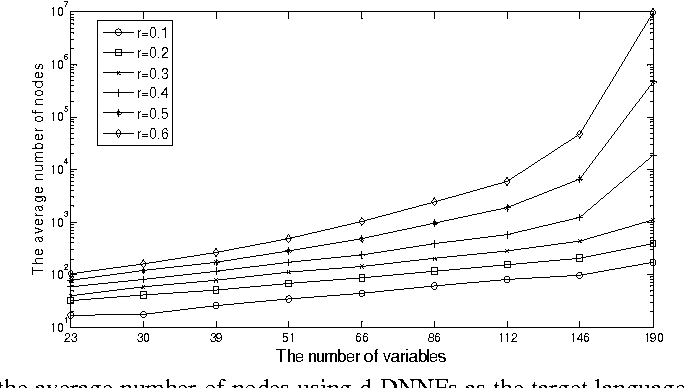Phase transitions in many complex combinational problems have been widely studied in the past decade. In this paper, we investigate phase transitions in the knowledge compilation empirically, where DFA, OBDD and d-DNNF are chosen as the target languages to compile random k-SAT instances. We perform intensive experiments to analyze the sizes of compilation results and draw the following conclusions: there exists an easy-hard-easy pattern in compilations; the peak point of sizes in the pattern is only related to the ratio of the number of clauses to that of variables when k is fixed, regardless of target languages; most sizes of compilation results increase exponentially with the number of variables growing, but there also exists a phase transition that separates a polynomial-increment region from the exponential-increment region; Moreover, we explain why the phase transition in compilations occurs by analyzing microstructures of DFAs, and conclude that a kind of solution interchangeability with more than 2 variables has a sharp transition near the peak point of the easy-hard-easy pattern, and thus it has a great impact on sizes of DFAs.

Click to Read Paper and Get Code
Backtracking is a basic strategy to solve constraint satisfaction problems (CSPs). A satisfiable CSP instance is backtrack-free if a solution can be found without encountering any dead-end during a backtracking search, implying that the instance is easy to solve. We prove an exact phase transition of backtrack-free search in some random CSPs, namely in Model RB and in Model RD. This is the first time an exact phase transition of backtrack-free search can be identified on some random CSPs. Our technical results also have interesting implications on the power of greedy algorithms, on the width of random hypergraphs and on the exact satisfiability threshold of random CSPs.

Click to Read Paper and Get Code
This paper analyzes the scaling window of a random CSP model (i.e. model RB) for which we can identify the threshold points exactly, denoted by $r_{cr}$ or $p_{cr}$. For this model, we establish the scaling window $W(n,\delta)=(r_{-}(n,\delta), r_{+}(n,\delta))$ such that the probability of a random instance being satisfiable is greater than $1-\delta$ for $r<r_{-}(n,\delta)$ and is less than $\delta$ for $r>r_{+}(n,\delta)$. Specifically, we obtain the following result $$W(n,\delta)=(r_{cr}-\Theta(\frac{1}{n^{1-\epsilon}\ln n}), \ r_{cr}+\Theta(\frac{1}{n\ln n})),$$ where $0\leq\epsilon<1$ is a constant. A similar result with respect to the other parameter $p$ is also obtained. Since the instances generated by model RB have been shown to be hard at the threshold, this is the first attempt, as far as we know, to analyze the scaling window of such a model with hard instances.

Click to Read Paper and Get Code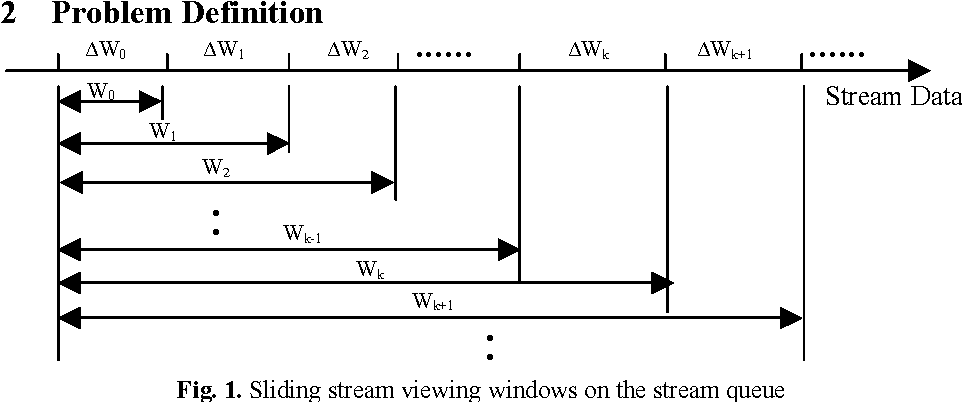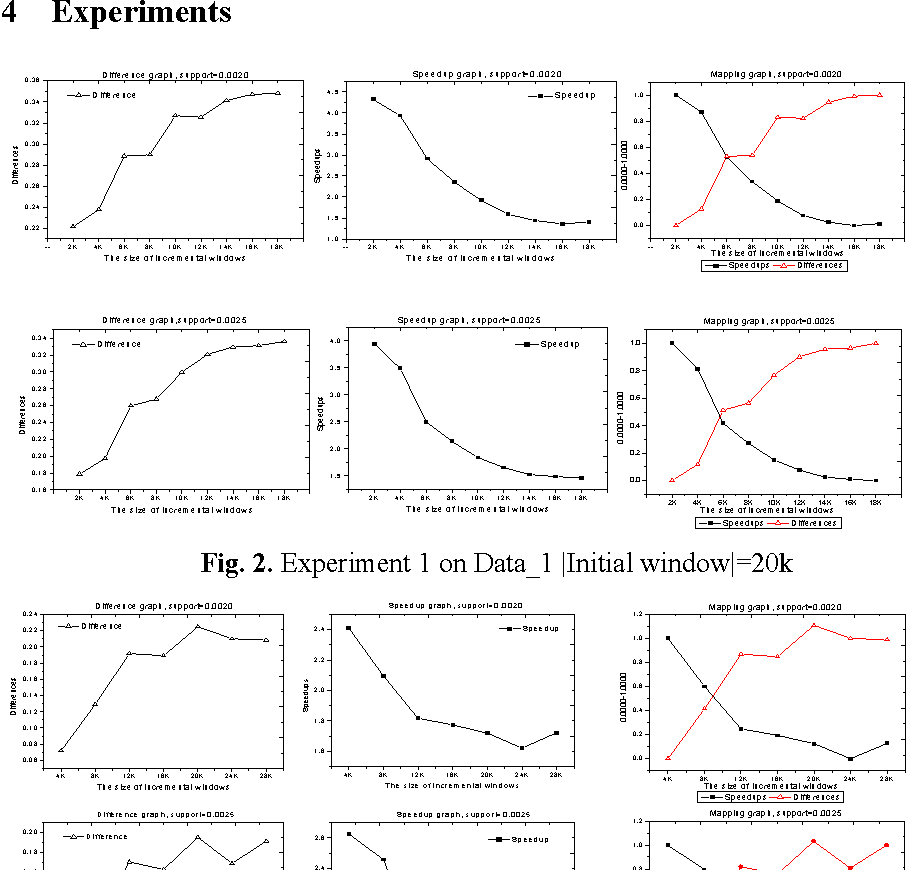In this paper, we first define a difference measure between the old and new sequential patterns of stream data, which is proved to be a distance. Then we propose an experimental method, called TPD (Tradeoff between Performance and Difference), to decide when to update the sequential patterns of stream data by making a tradeoff between the performance of increasingly updating algorithms and the difference of sequential patterns. The experiments for the incremental updating algorithm IUS on two data sets show that generally, as the size of incremental windows grows, the values of the speedup and the values of the difference will decrease and increase respectively. It is also shown experimentally that the incremental ratio determined by the TPD method does not monotonically increase or decrease but changes in a range between 20 and 30 percentage for the IUS algorithm.

* 12 pages, 5 figures
Click to Read Paper and Get Code
The limit behavior of inductive logic programs has not been explored, but when considering incremental or online inductive learning algorithms which usually run ongoingly, such behavior of the programs should be taken into account. An example is given to show that some inductive learning algorithm may not be correct in the long run if the limit behavior is not considered. An inductive logic program is convergent if given an increasing sequence of example sets, the program produces a corresponding sequence of the Horn logic programs which has the set-theoretic limit, and is limit-correct if the limit of the produced sequence of the Horn logic programs is correct with respect to the limit of the sequence of the example sets. It is shown that the GOLEM system is not limit-correct. Finally, a limit-correct inductive logic system, called the prioritized GOLEM system, is proposed as a solution.

* 9 pages. Welcome any comments to kexu@nlsde.buaa.edu.cn
Click to Read Paper and Get Code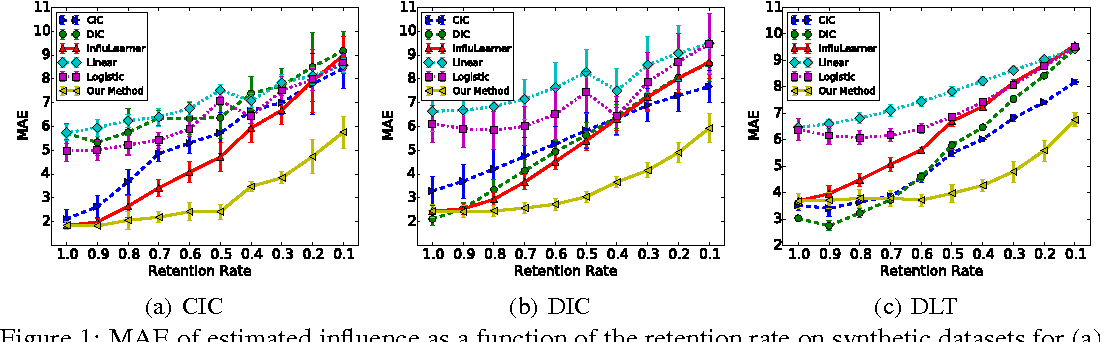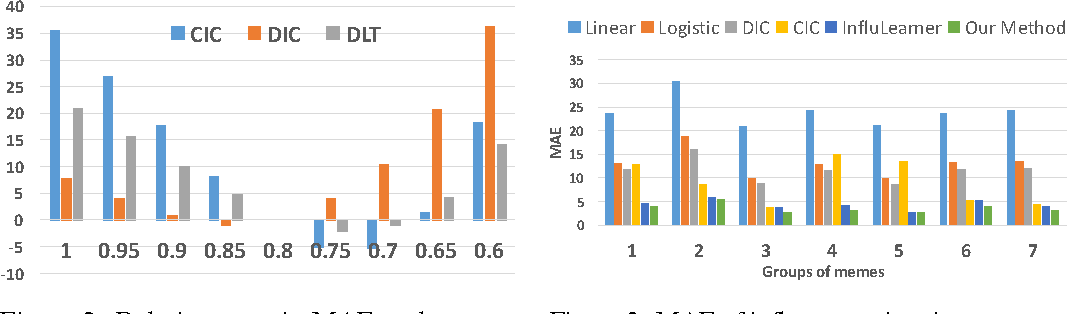We study the problem of learning influence functions under incomplete observations of node activations. Incomplete observations are a major concern as most (online and real-world) social networks are not fully observable. We establish both proper and improper PAC learnability of influence functions under randomly missing observations. Proper PAC learnability under the Discrete-Time Linear Threshold (DLT) and Discrete-Time Independent Cascade (DIC) models is established by reducing incomplete observations to complete observations in a modified graph. Our improper PAC learnability result applies for the DLT and DIC models as well as the Continuous-Time Independent Cascade (CIC) model. It is based on a parametrization in terms of reachability features, and also gives rise to an efficient and practical heuristic. Experiments on synthetic and real-world datasets demonstrate the ability of our method to compensate even for a fairly large fraction of missing observations.

* Full version of paper "Learning Influence Functions from Incomplete Observations" in NIPS16
Click to Read Paper and Get Code
Constraint satisfaction problems (CSPs) models many important intractable NP-hard problems such as propositional satisfiability problem (SAT). Algorithms with non-trivial upper bounds on running time for restricted SAT with bounded clause length k (k-SAT) can be classified into three styles: DPLL-like, PPSZ-like and Local Search, with local search algorithms having already been generalized to CSP with bounded constraint arity k (k-CSP). We generalize a DPLL-like algorithm in its simplest form and a PPSZ-like algorithm from k-SAT to k-CSP. As far as we know, this is the first attempt to use PPSZ-like strategy to solve k-CSP, and before little work has been focused on the DPLL-like or PPSZ-like strategies for k-CSP.

Click to Read Paper and Get Code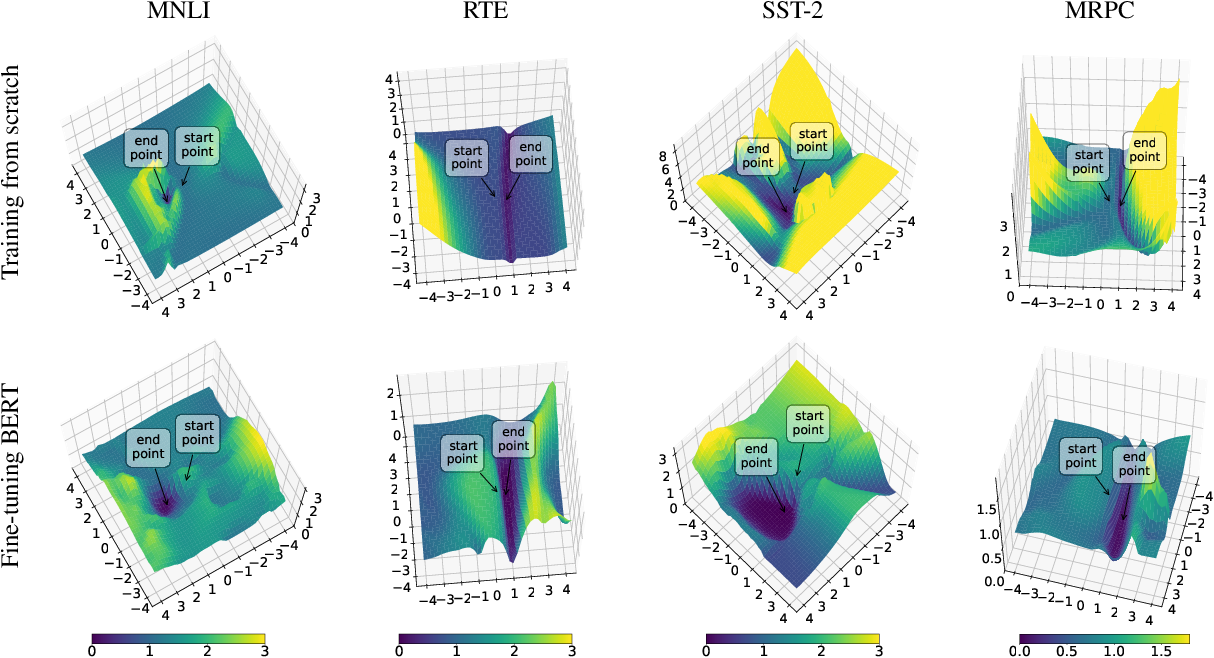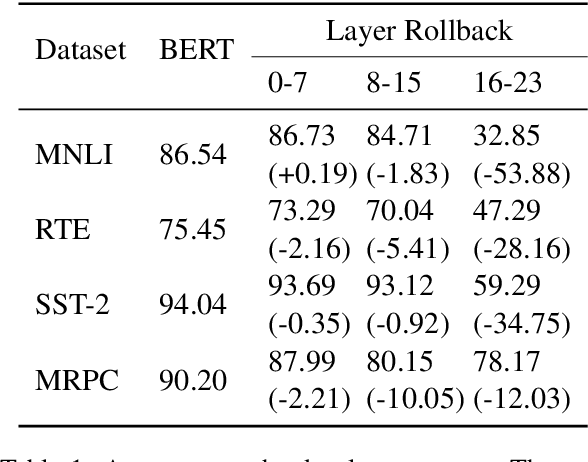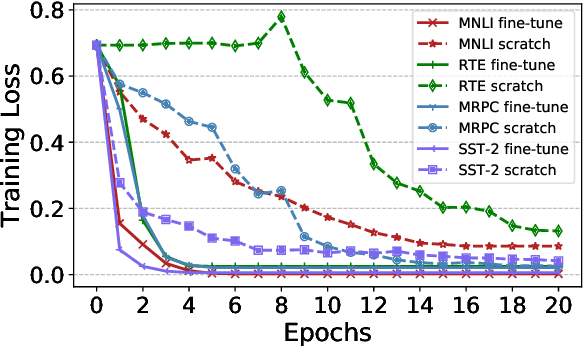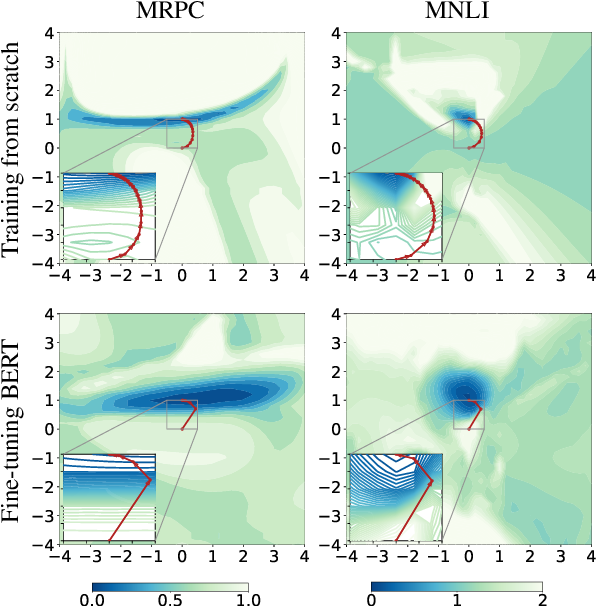Language model pre-training, such as BERT, has achieved remarkable results in many NLP tasks. However, it is unclear why the pre-training-then-fine-tuning paradigm can improve performance and generalization capability across different tasks. In this paper, we propose to visualize loss landscapes and optimization trajectories of fine-tuning BERT on specific datasets. First, we find that pre-training reaches a good initial point across downstream tasks, which leads to wider optima and easier optimization compared with training from scratch. We also demonstrate that the fine-tuning procedure is robust to overfitting, even though BERT is highly over-parameterized for downstream tasks. Second, the visualization results indicate that fine-tuning BERT tends to generalize better because of the flat and wide optima, and the consistency between the training loss surface and the generalization error surface. Third, the lower layers of BERT are more invariant during fine-tuning, which suggests that the layers that are close to input learn more transferable representations of language.

* Accepted by EMNLP-19
Click to Read Paper and Get Code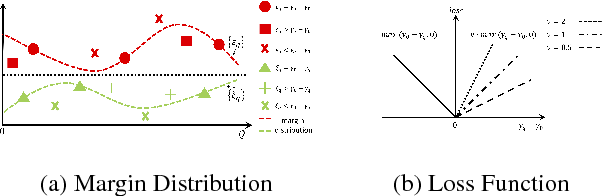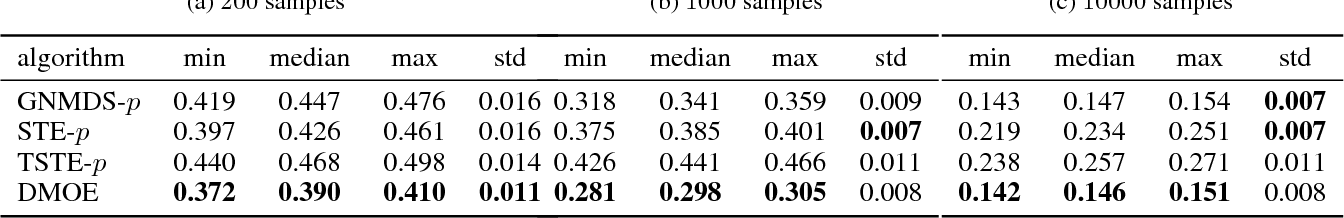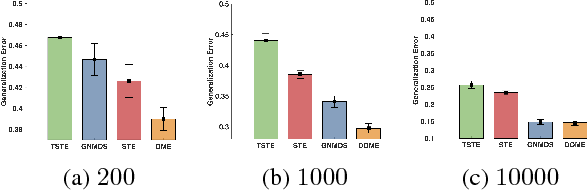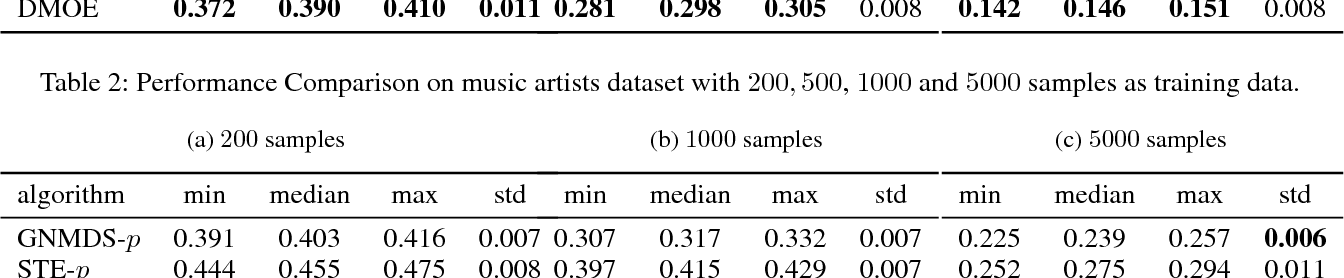In the absence of prior knowledge, ordinal embedding methods obtain new representation for items in a low-dimensional Euclidean space via a set of quadruple-wise comparisons. These ordinal comparisons often come from human annotators, and sufficient comparisons induce the success of classical approaches. However, collecting a large number of labeled data is known as a hard task, and most of the existing work pay little attention to the generalization ability with insufficient samples. Meanwhile, recent progress in large margin theory discloses that rather than just maximizing the minimum margin, both the margin mean and variance, which characterize the margin distribution, are more crucial to the overall generalization performance. To address the issue of insufficient training samples, we propose a margin distribution learning paradigm for ordinal embedding, entitled Distributional Margin based Ordinal Embedding (\textit{DMOE}). Precisely, we first define the margin for ordinal embedding problem. Secondly, we formulate a concise objective function which avoids maximizing margin mean and minimizing margin variance directly but exhibits the similar effect. Moreover, an Augmented Lagrange Multiplier based algorithm is customized to seek the optimal solution of \textit{DMOE} effectively. Experimental studies on both simulated and real-world datasets are provided to show the effectiveness of the proposed algorithm.

* Accepted by AAAI 2019
Click to Read Paper and Get Code